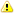## Data Types

Data type mapping:
 Oracle PostgreSQL 1 BFILE Pointer to binary file, ⇐ 4G VARCHAR(255) 2 BINARY_FLOAT 32-bit floating-point number REAL 3 BINARY_DOUBLE 64-bit floating-point number DOUBLE PRECISION 4 BLOB Binary large object, ⇐ 4GBYTEA 5 CHAR(n), CHARACTER(n) Fixed-length string, 1 ⇐ n ⇐ 2000 CHAR(n), CHARACTER(n) 6 CLOB Character large object, ⇐ 4GTEXT 7 DATE Date and time TIMESTAMP(0) 8 DECIMAL(p,s), DEC(p,s) Fixed-point number DECIMAL(p,s), DEC(p,s) 9 DOUBLE PRECISION Floating-point number DOUBLE PRECISION 10 FLOAT(p) Floating-point number DOUBLE PRECISION 11 INTEGER, INT 38 digits integer DECIMAL(38) 12 INTERVAL YEAR(p) TO MONTH Date interval INTERVAL YEAR TO MONTH 13 INTERVAL DAY(p) TO SECOND(s) Day and time interval INTERVAL DAY TO SECOND(s) 14 LONG Character data, ⇐ 2GTEXT 15 LONG RAW Binary data, ⇐ 2G BYTEA 16 NCHAR(n) Fixed-length UTF-8 string, 1 ⇐ n ⇐ 2000 CHAR(n)17 NCHAR VARYING(n) Varying-length UTF-8 string, 1 ⇐ n ⇐ 4000 VARCHAR(n)18 NCLOB Variable-length Unicode string, ⇐ 4GTEXT 19 NUMBER(p,0), NUMBER(p) 8-bit integer, 1 <= p < 3 SMALLINT 16-bit integer, 3 <= p < 5 SMALLINT 32-bit integer, 5 <= p < 9 INT 64-bit integer, 9 <= p < 19 BIGINT Fixed-point number, 19 <= p <= 38 DECIMAL(p) 20 NUMBER(p,s) Fixed-point number, s > 0 DECIMAL(p,s) 21 NUMBER, NUMBER(*) Floating-point number DOUBLE PRECISION 22 NUMERIC(p,s) Fixed-point number NUMERIC(p,s) 23 NVARCHAR2(n) Varying-length UTF-8 string, 1 ⇐ n ⇐ 4000 VARCHAR(n)24 RAW(n) Variable-length binary string, 1 ⇐ n ⇐ 2000 BYTEA 25 REAL Floating-point number DOUBLE PRECISION 26 ROWID Physical row address CHAR(10) 27 SMALLINT 38 digits integer DECIMAL(38) 28 TIMESTAMP(p) Date and time with fraction TIMESTAMP(p) 29 TIMESTAMP(p) WITH TIME ZONE Date and time with fraction and time zone TIMESTAMP(p) WITH TIME ZONE 30 UROWID(n) Logical row addresses, 1 ⇐ n ⇐ 4000 VARCHAR(n) 31 VARCHAR(n) Variable-length string, 1 ⇐ n ⇐ 4000 VARCHAR(n) 32 VARCHAR2(n) Variable-length string, 1 ⇐ n ⇐ 4000 VARCHAR(n) 33 XMLTYPE XML data XML